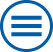## The group contributes to the following mathematical research topics of WIAS:

Large deviations

The theory of large deviations, a branch of probability theory, provides tools for the description of the asymptotic decay rate of a small probability, as a certain parameter diverges or shrinks to zero. Examples are large times, low temperatures, large numbers of stochastic quantities, or an approximation parameter. [>> more]

## Archive

### Mathematical research topics that are not treated at the moment but where the institute has expertise in

Interacting random systems

Large random interacting particle systems are mostly defined either by coupled systems of stochastic differential equations or via Hamiltonian functions. [>> more]